# Bresenham’s Algorithm for 3-D Line Drawing

Given two 3-D co-ordinates we need to find the points on the line joining them. All points have integer co-ordinates.

Examples:

```Input  : (-1, 1, 1), (5, 3, -1)
Output : (-1, 1, 1), (0, 1, 1), (1, 2, 0),
(2, 2, 0), (3, 2, 0), (4, 3, -1),
(5, 3, -1)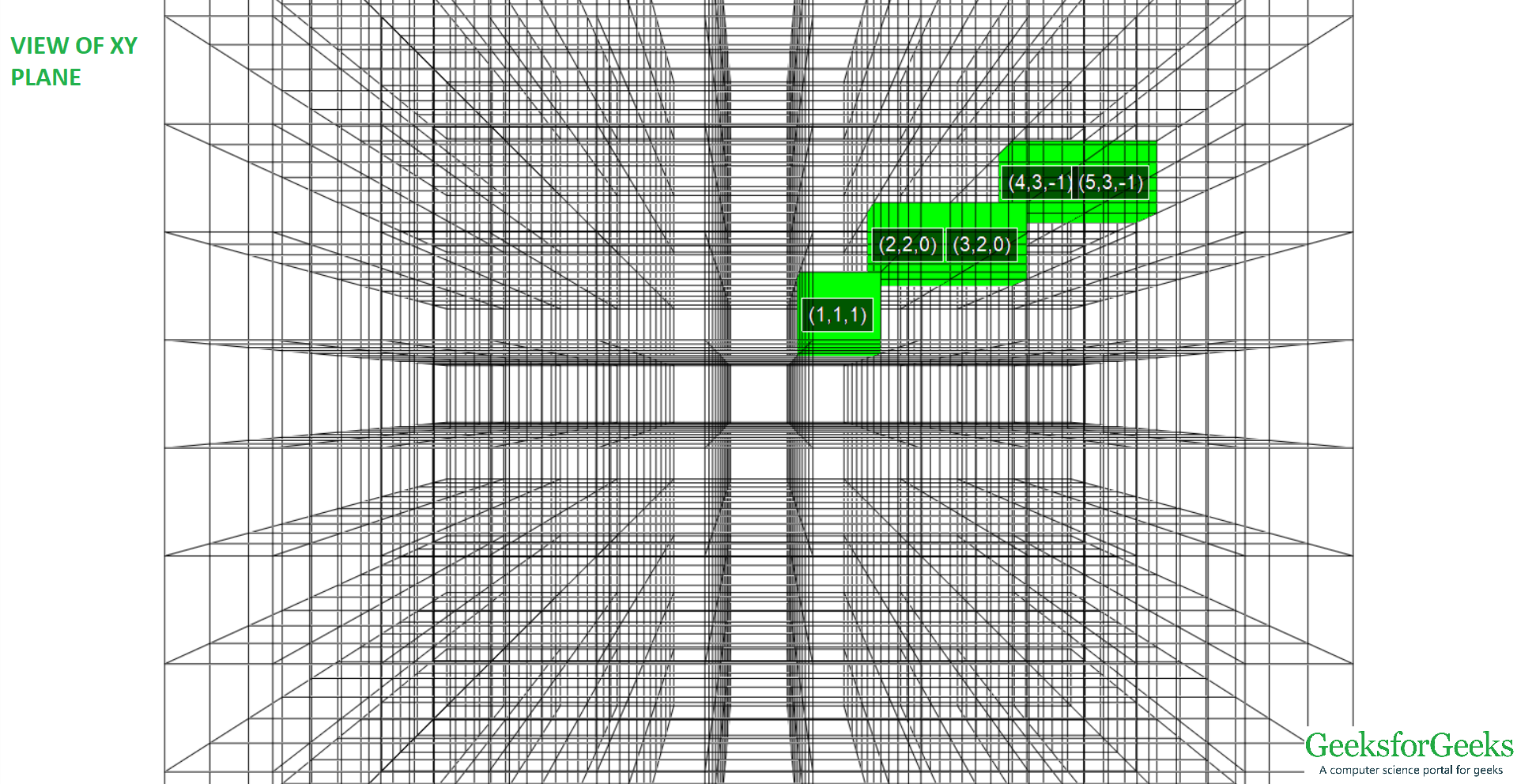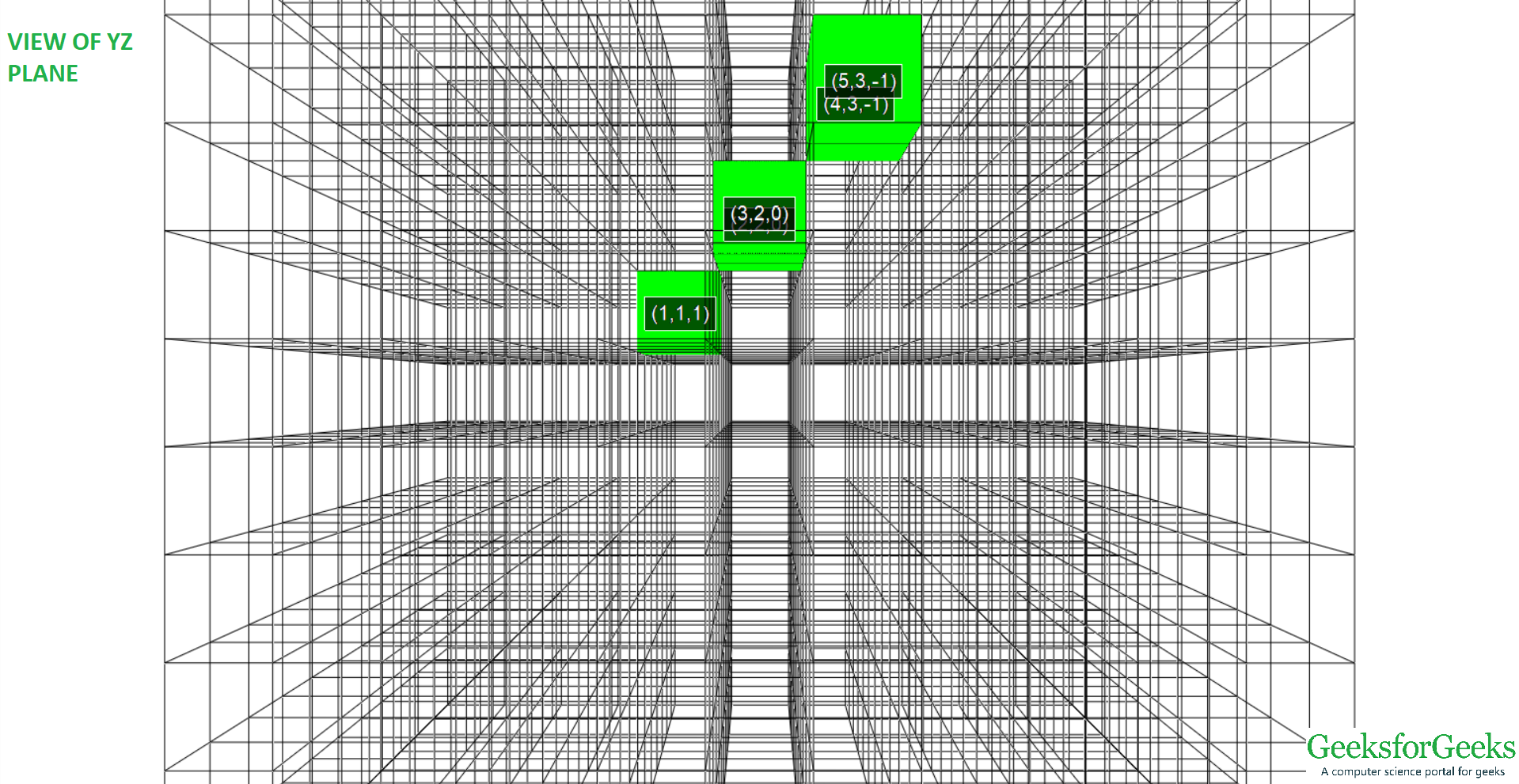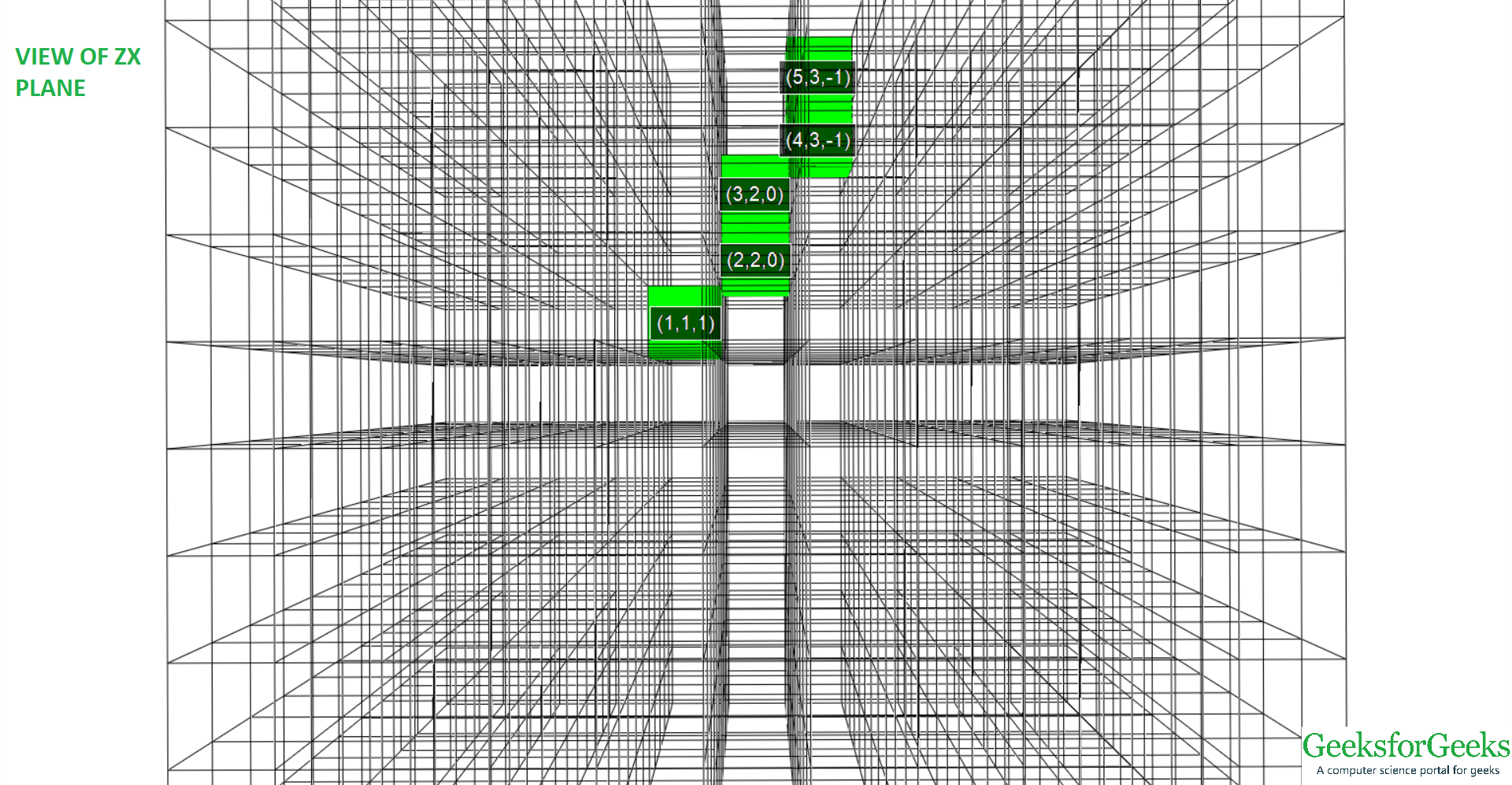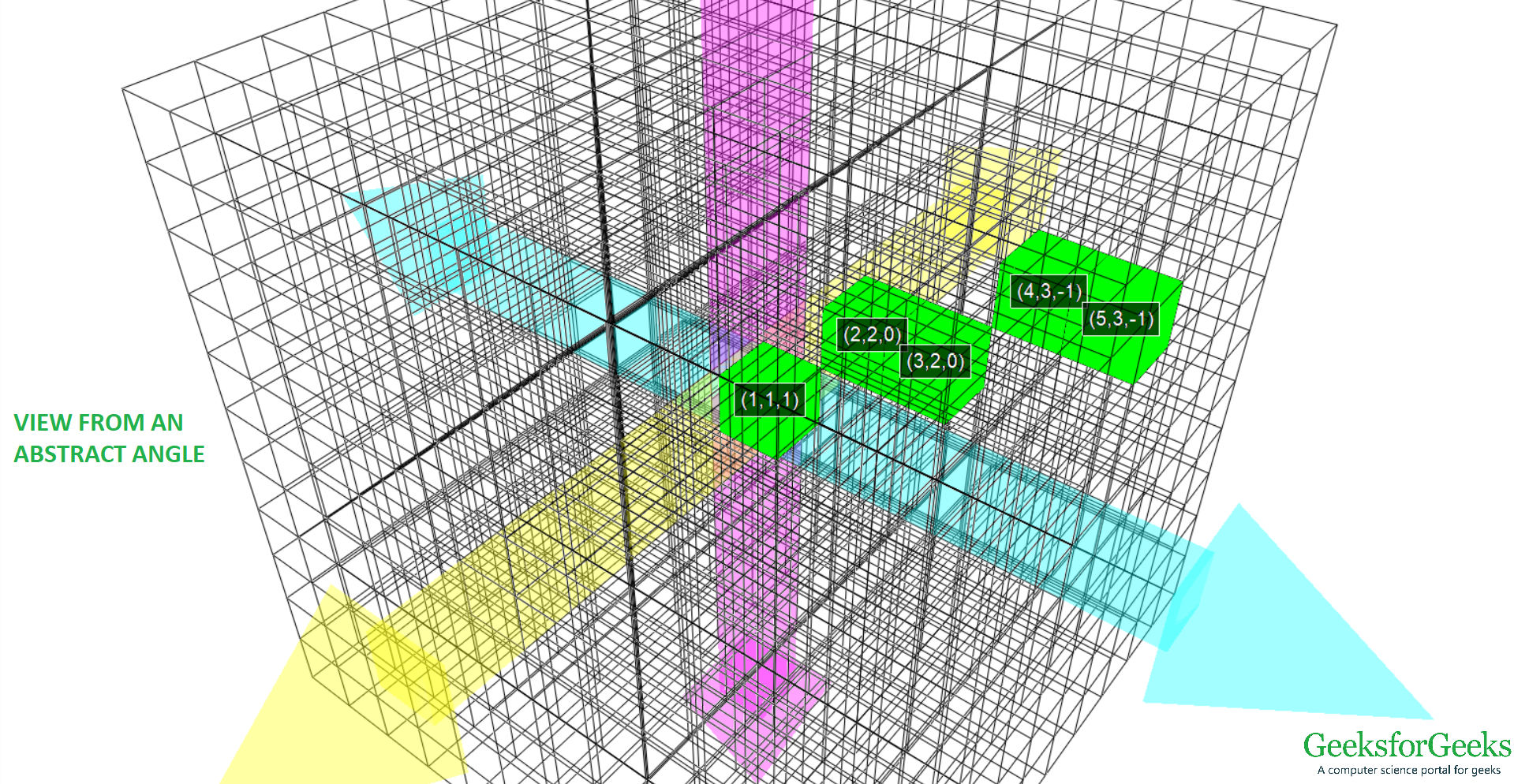Input  : (-7, 0, -3), (2, -5, -1)
Output : (-7, 0, -3), (-6, -1, -3), (-5, -1, -3),
(-4, -2, -2), (-3, -2, -2), (-2, -3, -2),
(-1, -3, -2), (0, -4, -1), (1, -4, -1),
(2, -5, -1)
```

Bresenham’s Algorithm is efficient as it avoids floating point arithmetic operations. As in the case of 2-D Line Drawing, we use a variable to store the slope-error i.e. the error in slope of the line being plotted from the actual geometric line. As soon as this slope-error exceeds the permissible value we modify the digital to negate the error.

The driving axis of the line to be plotted is the one along which the line travels the farthest i.e. the difference in axes co-ordinates is greatest. Thus the co-ordinate values increase linearly by 1 along the driving axis and the slope-error variable is used to determine the change in the co-ordinate values of the other axis.

In case of a 2-D line we use one slope-error variable but in case of a 3-D line we need two () of them for each of the non-driving axes. If current point is(x, y, z) and the driving axis is the positive X-axis, then the next pointcould be

• (x+1, y, z)
• (x+1, y+1, z)
• (x+1, y, z+1)
• (x+1, y+1, z+1)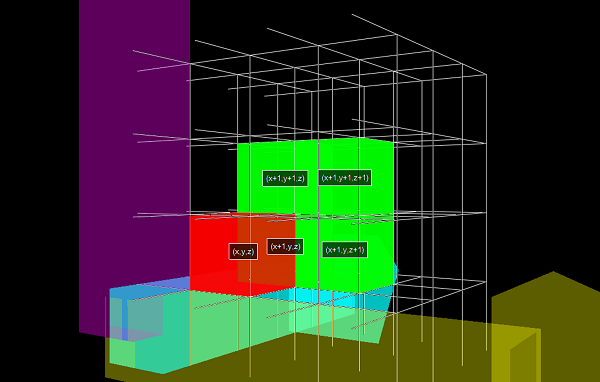The value of slope-error variables are determined according to the following equations:-The initial value of slope-error variables are given by the following equations:-Heredenote the difference in co-ordinates of the two end points along the X, Y, Z axes.

Algorithm:-

1. Input the two endpoints and store the initial point as2. Plot3. Calculate constantsand determine the driving axis by comparing
the absolute values ofIf abs() is maximum, then X-axis is the driving axis
If abs() is maximum, then Y-axis is the driving axis
If abs() is maximum, then Z-axis is the driving axis
4. Let’s suppose that X-axis is the driving axis, then5. At eachalong the line, starting at k = 0, check the following conditions
and determine the next point:-

• IfAND, then
plotand
set• Else IfAND, then
plotand
set• Else If, then
plotand
set• Else then
plotand
set>
6. Repeat step 5times

## Python3

 `# Python3 code for generating points on a 3-D line  ` `# using Bresenham's Algorithm ` ` `  `def` `Bresenham3D(x1, y1, z1, x2, y2, z2): ` `    ``ListOfPoints ``=` `[] ` `    ``ListOfPoints.append((x1, y1, z1)) ` `    ``dx ``=` `abs``(x2 ``-` `x1) ` `    ``dy ``=` `abs``(y2 ``-` `y1) ` `    ``dz ``=` `abs``(z2 ``-` `z1) ` `    ``if` `(x2 > x1): ` `        ``xs ``=` `1` `    ``else``: ` `        ``xs ``=` `-``1` `    ``if` `(y2 > y1): ` `        ``ys ``=` `1` `    ``else``: ` `        ``ys ``=` `-``1` `    ``if` `(z2 > z1): ` `        ``zs ``=` `1` `    ``else``: ` `        ``zs ``=` `-``1` ` `  `    ``# Driving axis is X-axis" ` `    ``if` `(dx >``=` `dy ``and` `dx >``=` `dz):         ` `        ``p1 ``=` `2` `*` `dy ``-` `dx ` `        ``p2 ``=` `2` `*` `dz ``-` `dx ` `        ``while` `(x1 !``=` `x2): ` `            ``x1 ``+``=` `xs ` `            ``if` `(p1 >``=` `0``): ` `                ``y1 ``+``=` `ys ` `                ``p1 ``-``=` `2` `*` `dx ` `            ``if` `(p2 >``=` `0``): ` `                ``z1 ``+``=` `zs ` `                ``p2 ``-``=` `2` `*` `dx ` `            ``p1 ``+``=` `2` `*` `dy ` `            ``p2 ``+``=` `2` `*` `dz ` `            ``ListOfPoints.append((x1, y1, z1)) ` ` `  `    ``# Driving axis is Y-axis" ` `    ``elif` `(dy >``=` `dx ``and` `dy >``=` `dz):        ` `        ``p1 ``=` `2` `*` `dx ``-` `dy ` `        ``p2 ``=` `2` `*` `dz ``-` `dy ` `        ``while` `(y1 !``=` `y2): ` `            ``y1 ``+``=` `ys ` `            ``if` `(p1 >``=` `0``): ` `                ``x1 ``+``=` `xs ` `                ``p1 ``-``=` `2` `*` `dy ` `            ``if` `(p2 >``=` `0``): ` `                ``z1 ``+``=` `zs ` `                ``p2 ``-``=` `2` `*` `dy ` `            ``p1 ``+``=` `2` `*` `dx ` `            ``p2 ``+``=` `2` `*` `dz ` `            ``ListOfPoints.append((x1, y1, z1)) ` ` `  `    ``# Driving axis is Z-axis" ` `    ``else``:         ` `        ``p1 ``=` `2` `*` `dy ``-` `dz ` `        ``p2 ``=` `2` `*` `dx ``-` `dz ` `        ``while` `(z1 !``=` `z2): ` `            ``z1 ``+``=` `zs ` `            ``if` `(p1 >``=` `0``): ` `                ``y1 ``+``=` `ys ` `                ``p1 ``-``=` `2` `*` `dz ` `            ``if` `(p2 >``=` `0``): ` `                ``x1 ``+``=` `xs ` `                ``p2 ``-``=` `2` `*` `dz ` `            ``p1 ``+``=` `2` `*` `dy ` `            ``p2 ``+``=` `2` `*` `dx ` `            ``ListOfPoints.append((x1, y1, z1)) ` `    ``return` `ListOfPoints ` ` `  ` `  `def` `main(): ` `    ``(x1, y1, z1) ``=` `(``-``1``, ``1``, ``1``) ` `    ``(x2, y2, z2) ``=` `(``5``, ``3``, ``-``1``) ` `    ``ListOfPoints ``=` `Bresenham3D(x1, y1, z1, x2, y2, z2) ` `    ``print``(ListOfPoints) ` ` `  `main() `

Output:

```[(-1, 1, 1), (0, 1, 1), (1, 2, 0), (2, 2, 0), (3, 2, 0), (4, 3, -1), (5, 3, -1)]
```

Attention reader! Don’t stop learning now. Get hold of all the important DSA concepts with the DSA Self Paced Course at a student-friendly price and become industry ready.

My Personal Notes arrow_drop_upCheck out this Author's contributed articles.

If you like GeeksforGeeks and would like to contribute, you can also write an article using contribute.geeksforgeeks.org or mail your article to contribute@geeksforgeeks.org. See your article appearing on the GeeksforGeeks main page and help other Geeks.

Please Improve this article if you find anything incorrect by clicking on the "Improve Article" button below.